Courses

# Chapter Notes - Understanding Elementary Shapes Class 6 Notes | EduRev

## Class 6 : Chapter Notes - Understanding Elementary Shapes Class 6 Notes | EduRev

The document Chapter Notes - Understanding Elementary Shapes Class 6 Notes | EduRev is a part of the Class 6 Course Mathematics (Maths) Class 6.
All you need of Class 6 at this link: Class 6

Line and Angles: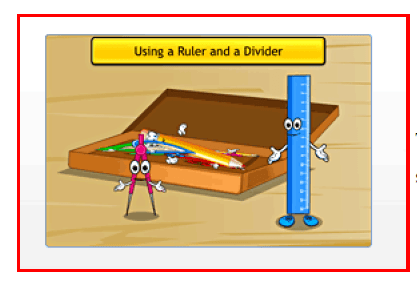The distance between the endpoints of a line segment is the length of the line segment.

Length of a line segment:
The distance between the endpoints of a line segment is the length of the line segment. The length of a line segment can be measured accurately using a ruler and a divider.

Complete angle:
An angle of measure 360° is called a complete angle.
One quadrant = ¼ (Complete angle) = 1/4 x 360°90° = Two quadrants = 1/2
(Complete angle) = 1/2 x 360° = 180°
Three quadrants = 3/4 (Complete angle) = 3/4 x 360° = 270°

Right angle:
An angle that measures 90° is called a right angle. A right angle makes a quarter revolutions.

Straight angle:
An angle that measures 180° is called a straight angle. A straight angle makes a half revolution.

Acute angle:
An angle that measures less than 90° is called an acute angle.

Obtuse angle:
An angle that measures more than 90° and less than 180° is called an obtuse angle.

Reflex angle:
An angle that measures more than 180° is called a reflex angle.

Intersecting lines:
Two lines that meet each other at a single point are called intersecting lines.

Perpendicular lines:
Two lines that intersect each other at right angles are said to be perpendicular to each other.

Bisector of a line segment:
A bisector of a line segment is a line that divides the line segment into two equal parts.

Perpendicular bisector of a line segment:
The perpendicular line that divides a line segment into two equal parts is called the perpendicular bisector of the line segment.

Two Dimensional Figures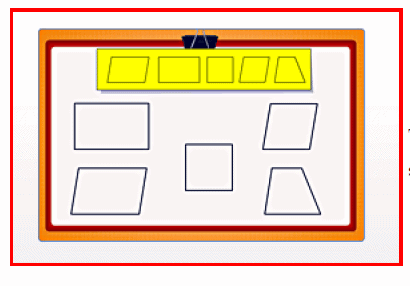The closed figure formed by joining three line segments end-to-end is called a triangle. Each line segment forms a side of the triangle.

Scalene Triangle:
A triangle is called a scalene triangle if all the three sides are of unequal length.

Isosceles Triangles:
A triangle is called an isosceles triangle if two of its sides are of equal length.

Equilateral Triangle:
A triangle is said to be an equilateral triangle if the lengths of all of its sides are equal.

Acute-Angled Triangle:
If all the angles of a triangle are less than 90°, then the triangle is called an acute-angled triangle.

Right-Angled triangle:
If one of the angles in a triangle is a right angle, then the triangle is called a right-angled triangle.

Obtuse-Angled Triangle:
If one of the angles in a triangle is an obtuse angle, then the triangle is called an obtuse-angled triangle.

Parallelogram:
A parallelogram is a four-sided figure in which the opposite sides are parallel to each other and are of equal length. In a parallelogram, the diagonals need not be equal in length.

Rectangle:
A rectangle is a type of parallelogram that has opposite sides equal in length and parallel to each other. Its diagonals are equal in length. A rectangle has four right angles.

Square:
A square is a type of parallelogram in which all the four sides are equal in length. Its diagonals are equal in length. A square has four right angles.

Rhombus:
In a rhombus, all the sides are equal in length, and the opposite sides are parallel to each other. Its diagonals are not equal in length. Also, the opposite angles are equal to each other.

Trapezium:
A trapezium has one pair of sides parallel to each other. The other two sides are not parallel to each other.

Polygon:
A polygon is a closed figure with three or more than three sides.

Three Dimensional Shapes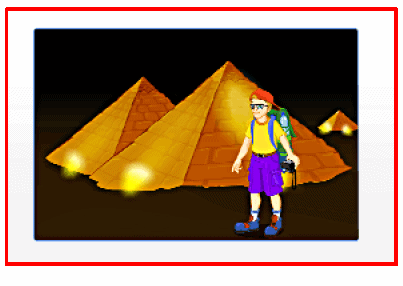Solid figures have three dimensions - length, breadth and height.
Eg: A ball, a brick, an ice cream cone and a can.

Face
The flat surface of a solid shape is called a face.

Edge
An edge is a line segment two faces of a solid shape meet.

Vertex
A vertex of a solid shape is a point where three or more edges meet. A cuboid has 6 faces, 12 edges and 8 vertices. Prisms and pyramids are named after their bases. The base of a prism can be of any polygonal shape.
There are 5 faces, 9 edges and 6 vertices in a

Triangular prism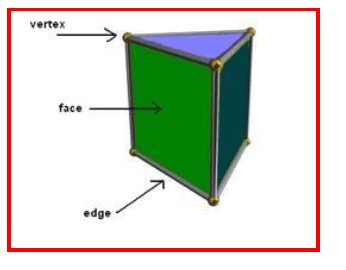There are 4 faces, 6 edges and 4 vertices in a triangular pyramid.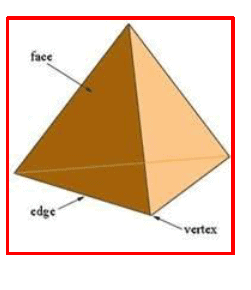Offer running on EduRev: Apply code STAYHOME200 to get INR 200 off on our premium plan EduRev Infinity!

## Mathematics (Maths) Class 6

185 videos|229 docs|43 tests

,

,

,

,

,

,

,

,

,

,

,

,

,

,

,

,

,

,

,

,

,

;